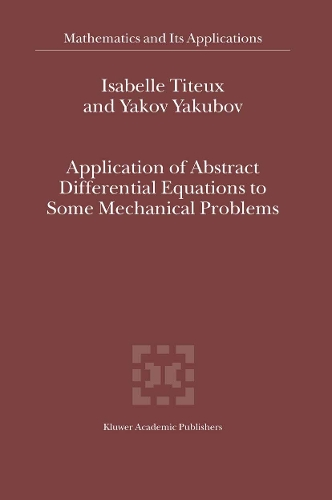•# Application of Abstract Differential Equations to Some Mechanical Problems - Mathematics and Its Applications 558 (Paperback)

(author), (author)
£69.99
Paperback 209 Pages / Published: 30/09/2012
• We can order this

Usually dispatched within 3 weeks

PREFACE The theory of differential-operator equations has been described in various monographs, but the initial physical problem which leads to these equations is often hidden. When the physical problem is studied, the mathematical proofs are either not given or are quickly explained. In this book, we give a systematic treatment of the partial differential equations which arise in elastostatic problems. In particular, we study problems which are obtained from asymptotic expansion with two scales. Here the methods of operator pencils and differential-operator equations are used. This book is intended for scientists and graduate students in Functional Analy sis, Differential Equations, Equations of Mathematical Physics, and related topics. It would undoubtedly be very useful for mechanics and theoretical physicists. We would like to thank Professors S. Yakubov and S. Kamin for helpfull dis cussions of some parts of the book. The work on the book was also partially supported by the European Community Program RTN-HPRN-CT-2002-00274. xiii INTRODUCTION In first two sections of the introduction, a classical mathematical problem will be exposed: the Laplace problem. The domain of definition will be, on the first time, an infinite strip and on the second time, a sector. To solve this problem, a well known separation of variables method will be used. In this way, the structure of the solution can be explicitly found. For more details about the separation of variables method exposed in this part, the reader can refer to, for example, the book by D. Leguillon and E. Sanchez-Palencia [LS].

Publisher: Springer
ISBN: 9789401037846
Number of pages: 209
Weight: 378 g
Dimensions: 240 x 160 x 12 mm
Edition: Softcover reprint of the original 1st ed. 200